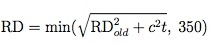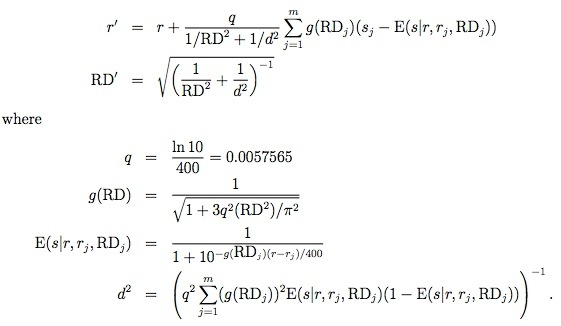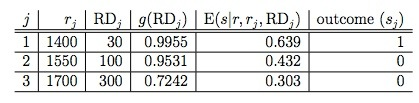# Glicko Rating System: A simple example using Clojure

A couple of weeks ago I wrote about the Elo Rating system and when reading more about it I learnt that one of its weaknesses is that it doesn't take into account the reliability of a players' rating.

For example, a player may not have played for a long time. When they next play a match we shouldn't assume that the accuracy of that rating is the same as for another player with the same rating but who plays regularly.

Mark Glickman wrote the Glicko Rating System to take the uncertainty into account by introducing a 'ratings deviation' (RD). A low RD indicates that a player competes frequently and a higher RD indicates that they don't.

One other difference between Glicko and Elo is the following:

It is interesting to note that, in the Glicko system, rating changes are not balanced as they usually are in the Elo system. If one player's rating increases by x, the opponent's rating does not usually decrease by x as in the Elo system. In fact, in the Glicko system, the amount by which the opponent's rating decreases is governed by both players' RD's.

The RD value effectively tells us the range in which the player's actual rating probably exists. i.e. a 95% confidence interval.

e.g. if a player has a rating of 1850 and a RD of 50 then the interval is 1750 - 1950 or (Rating - 2*RD) - (Rating + 2*RD)

The algorithm has 2 steps:

1. Determine a rating and RD for each player at the onset of the rating period. If the player is unrated use a value of 1500 and RD of 350. If they do have a rating we'll calculate the new RD from the old RD using this formula:where:
• t is the number of rating periods since last competition (e.g., if the player competed in the most recent rating period, t = 1)
• c is a constant that governs the increase in uncertainty over time.
2. Update each players rating and RD separately using the following formula:where:
• r is the player's pre-period rating
• RD is the player's pre-period ratings deviation
• r1, r2,...,rm are the pre-period ratings of their opponents
• RD1, RD2,...,RDm are the pre-period ratings deviations of their opponents
• s1, s2,...,2m are the scores against the opponents. 1 is a win, 1/2 is a draw, 0 is a defeat.
• r' is the player's post-period rating
• RD' is the player's post-period ratings deviation

The paper provides an example to follow and includes the intermediate workings which made it easier to build the algorithm one function at a time.

The q function was the simplest to implement so I created that and the g function at the same time:

``````
(ns ranking-algorithms.glicko
(:require [clojure.math.numeric-tower :as math]))

(def q
(/ (java.lang.Math/log 10) 400))

(defn g [rd]
(/ 1
(java.lang.Math/sqrt (+ 1
(/ (* 3 (math/expt q 2) (math/expt rd 2))
(math/expt ( . Math PI) 2))))))
``````

We can use the following table to check we get the right results when we call it.:``````
> (g 30)
0.9954980060779481
> (g 100)
0.953148974234587
> (g 300)
0.7242354637384434
``````

The next easiest function to write was the E function:

``````
(defn e [rating opponent-rating opponent-rd]
(/ 1
(+ 1
(math/expt 10 (/ (* (- (g opponent-rd))
(- rating opponent-rating))
400)))))
``````

And if we test that assuming that we have a rating of 1500 with a RD of 200:

``````
> (e 1500 1400 30)
0.639467736007921
> (e 1500 1550 100)
0.43184235355955686
> (e 1500 1700 300)
0.30284072524764
``````

Finally we need to write the d2 supporting function:

``````
(defn d2 [opponents]
(/ 1  (* (math/expt q 2)
(reduce process-opponent 0 opponents))))

(defn process-opponent [total opponent]
(let [{:keys [g e]} opponent]
(+ total (* (math/expt g 2) e (- 1 e)))))
``````

In this function we need to sum a combination of the g and e values we calculated earlier for each opponent so we can use a reduce over a collection of those values for each opponent to do that:

``````
> (d2 [{:g (g 30) :e (e 1500 1400 30)}
{:g (g 100) :e (e 1500 1550 100)}
{:g (g 300) :e (e 1500 1700 300)}])
53685.74290197874
``````

I get a slightly different value for this function which I think is because I didn't round the intermediate values to 2 decimal places as the example does.

Now we can introduce the r' function which returns our ranking after taking the matches against these opponents into account:

``````
(defn update-ranking [ranking-delta opponent]
(let [{:keys [ranking opponent-ranking opponent-ranking-rd score]} opponent]
(+ ranking-delta
(* (g opponent-ranking-rd)
(- score (e ranking opponent-ranking opponent-ranking-rd))))))

(defn g-and-e
[ranking {o-rd :opponent-ranking-rd o-ranking :opponent-ranking}]
{:g (g o-rd) :e (e ranking o-ranking o-rd)})

(defn ranking-after-round
[{ ranking :ranking rd :ranking-rd opponents :opponents}]
(+ ranking
(* (/ q
(+ (/ 1 (math/expt rd 2))
(/ 1 (d2 (map (partial g-and-e ranking) opponents)))))
(reduce update-ranking 0 (map #(assoc-in % [:ranking] ranking) opponents)))))
``````

One thing I wasn't sure about here was the use of partial which is a bit of a Haskell idiom. I'm not sure what the favoured approach is in Clojure land yet.

If we execute that function we get the expected result:

``````
> (ranking-after-round { :ranking 1500
:ranking-rd 200
:opponents[{:opponent-ranking 1400 :opponent-ranking-rd 30 :score 1}
{:opponent-ranking 1550 :opponent-ranking-rd 100 :score 0}
{:opponent-ranking 1700 :opponent-ranking-rd 300 :score 0}]})
1464.1064627569112
``````

The only function missing now is RD' which returns our RD after taking these matches into account:

``````
(defn rd-after-round
[{ ranking :ranking rd :ranking-rd opponents :opponents}]
(java.lang.Math/sqrt (/ 1 (+ (/ 1 (math/expt rd 2)
)
(/ 1 (d2 (map (partial g-and-e ranking) opponents)))))))
``````

If we execute that function we get the expected result and we're done!

``````
> (rd-after-round { :ranking 1500
:ranking-rd 200
:opponents[{:opponent-ranking 1400 :opponent-ranking-rd 30 :score 1}
{:opponent-ranking 1550 :opponent-ranking-rd 100 :score 0}
{:opponent-ranking 1700 :opponent-ranking-rd 300 :score 0}]})
151.39890244796933
``````

The next step is to run this algorithm against the football data and see if its results differ to the ones I got with the Elo algorithm.

I'm still not quite sure what I should set the rating period to. My initial thinking was that the rating period could be a season but that would mean that a team's rating only really makes sense after a few seasons of matches.

The code is on github if you want to play with it and if you have any suggestions on how to make the code more idiomatic I'd love to hear them.